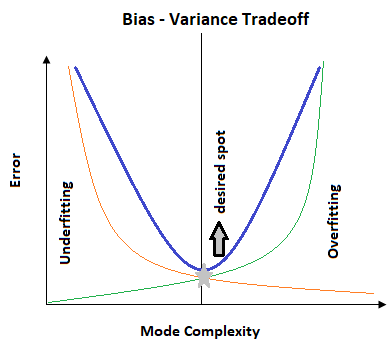# Day 48(ML) — Hyperparameters tuning in Machine Learning ModelsPic from shopify

Before tuning the ML model, it is essential to understand the performance of the model and how to enhance it. To evaluate the performance, different types of metrics are used depends on the type of the problem whether it is regression or classification. If the parameters of the model are tuned with the training dataset, it would result in overfitting. As the model is 100% accurate in train but fails to produce desired outcomes in the validation.

In order to design a generalized model, the entire dataset is split into train, validation and test. Using the training set, the model is fit and with the help of validation dataset, the hyperparameters are fine-tuned to generate an efficient model. Once all the parameters are finalised, then the test dataset is used for the prediction. Based on the predicted values of the test, it is decided whether the model is meeting the expectation or overfit or underfit. The testing set is always equal to the production data and the model should never be tweaked based on the test data, which has to be always treated as unseen production samples.fig1:- shows the bias-variance tradeoff curve

Any ML model should converge to the desired spot which has balanced bias and variance. After that point, the model starts to perform poorly on the test dataset even though it produces higher accuracy with the training dataset.

Occam’s Razor simplicity:- The above statement is basically a version of Occam’s theorem which states that a model should neither be too simple nor complex. But it should be simpler.

There are different points one needs to consider in order to achieve an effective model.

1. Choosing the right attributes:- The independent variables should be chosen in such a manner that they are excellent predictors of the target variable. By following appropriate steps in EDA, the features can be selected.

Let’s focus more on the fine-tuning the hyperparameters. The different steps involved during this process are:-

step1: Selecting the appropriate model type (i.e) either classification or regression.

step2: Identification of corresponding parameters. get_params() in python provides the list of model parameters.

step3: Decide the method for searching or sampling the hyperparameter space. Here either GridSearchCV or RandomizedSearchCV is used.

step4: Determine the cross-validation scheme to ensure the model will generalize.

step5: Finalise the scoring function that can be used to evaluate the performance of the model.

GridSearchCV: Here a grid is created which contains the list of hyperparameters and the values corresponding to it. For each combination of the hyperparameter values, the cross-validation score is calculated. The hyperparameters which produce the highest score will be used for subsequent processing. The challenge of the grid search is the user has to define the range of values for the continuous hyperparameters. To cite an example, in case of ridge or lasso, lambda is a hyperparameter and the values defined in the user’s list may not be the accurate one. Because the best one may not even be listed out.

RandomizedSearchCV: This approach basically works on the principle of random sampling. Rather than trying out for all the combinations of hyperparameter values given, it randomly samples the parameter values and applies it to the model. Sampling will be done without replacement when the values for the continuous parameters are given as a list, whereas, sampling with replacement is performed if the parameter values are given as distributions. The sampling method is proved to be a better performer than the grid search. This is motivated by the concept that not all hyperparameters are equally important.

Both the search processes are aimed at continuous hyperparameters, as the possible range of values for a continuous variable is enormous when compared to the discrete one.

The entire code can be found in the GitHub repository.

Recommended Reading:

https://papers.nips.cc/paper/4443-algorithms-for-hyper-parameter-optimization.pdf

https://towardsdatascience.com/machine-learning-gridsearchcv-randomizedsearchcv-d36b89231b10

AI Enthusiast | Blogger✍